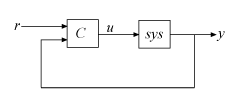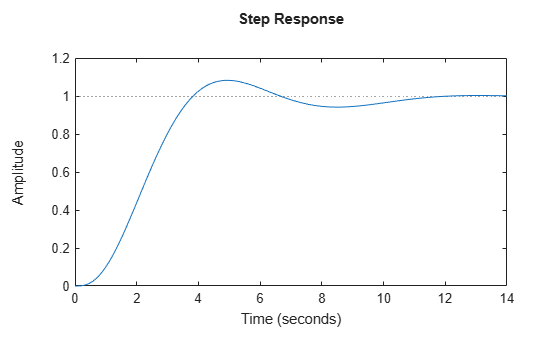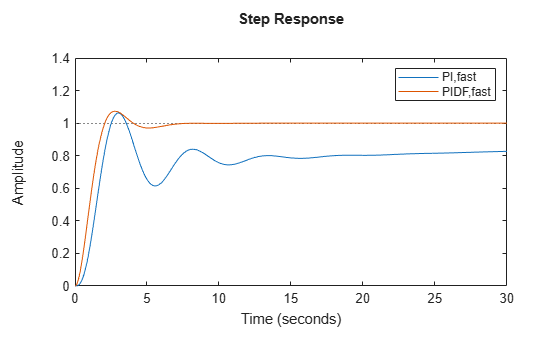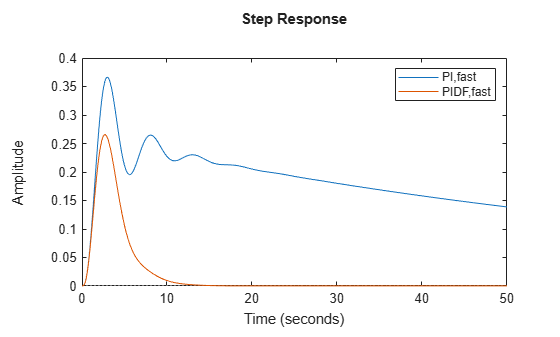Documentation

# pidtune

PID tuning algorithm for linear plant model

## Syntax

```C = pidtune(sys,type) C = pidtune(sys,C0) C = pidtune(sys,type,wc) C = pidtune(sys,C0,wc) C = pidtune(sys,...,opts) [C,info] = pidtune(...) ```

## Description

`C = pidtune(sys,type)` designs a PID controller of type `type` for the plant `sys`. If `type` specifies a one-degree-of-freedom (1-DOF) PID controller, then the controller is designed for the unit feedback loop as illustrated:If `type` specifies a two-degree-of-freedom (2-DOF) PID controller, then `pidtune` designs a 2-DOF controller as in the feedback loop of this illustration:`pidtune` tunes the parameters of the PID controller `C` to balance performance (response time) and robustness (stability margins).

`C = pidtune(sys,C0)` designs a controller of the same type and form as the controller `C0`. If `sys` and `C0` are discrete-time models, `C` has the same discrete integrator formulas as `C0`.

`C = pidtune(sys,type,wc)` and ```C = pidtune(sys,C0,wc)``` specify a target value `wc` for the first 0 dB gain crossover frequency of the open-loop response.

`C = pidtune(sys,...,opts)` uses additional tuning options, such as the target phase margin. Use `pidtuneOptions` to specify the option set `opts`.

```[C,info] = pidtune(...)``` returns the data structure `info`, which contains information about closed-loop stability, the selected open-loop gain crossover frequency, and the actual phase margin.

## Input Arguments

 `sys` Single-input, single-output dynamic system model of the plant for controller design. `sys` can be: Any type of SISO dynamic system model, including Numeric LTI models and identified models. If `sys` is a tunable or uncertain model, `pidtune` designs a controller for the current or nominal value of `sys`.A continuous- or discrete-time model.Stable, unstable, or integrating. A plant with unstable poles, however, might not be stabilizable under PID control.A model that includes any type of time delay. A plant with long time delays, however, might not achieve adequate performance under PID control.An array of plant models. If `sys` is an array, `pidtune` designs a separate controller for each plant in the array. If the plant has unstable poles, and `sys` is one of the following: A `frd` modelA `ss` model with internal time delays that cannot be converted to I/O delays you must use `pidtuneOptions` to specify the number of unstable poles in the plant, if any.

`type`

Controller type of the controller to design, specified as a character vector. The term controller type refers to which terms are present in the controller action. For example, a PI controller has only a proportional and an integral term, while a PIDF controller contains proportional, integrator, and filtered derivative terms. `type` can take the values summarized below. For more detailed information about these controller types, see PID Controller Types for Tuning

#### 1-DOF Controllers

• `'P'` — Proportional only

• `'I'` — Integral only

• `'PI'` — Proportional and integral

• `'PD'` — Proportional and derivative

• `'PDF'` — Proportional and derivative with first-order filter on derivative term

• `'PID'` — Proportional, integral, and derivative

• `'PIDF'` — Proportional, integral, and derivative with first-order filter on derivative term

#### 2-DOF Controllers

• `'PI2'` — 2-DOF proportional and integral

• `'PD2'` — 2-DOF proportional and derivative

• `'PDF2'` — 2-DOF proportional and derivative with first-order filter on derivative term

• `'PID2'` — 2-DOF proportional, integral, and derivative

• `'PIDF2'` — 2-DOF proportional, integral, and derivative with first-order filter on derivative term

#### 2-DOF Controllers with Fixed Setpoint Weights

• `'I-PD'` — 2-DOF PID with b = 0, c = 0

• `'I-PDF'` — 2-DOF PIDF with b = 0, c = 0

• `'ID-P'` — 2-DOF PID with b = 0, c = 1

• `'IDF-P'` — 2-DOF PIDF with b = 0, c = 1

• `'PI-D'` — 2-DOF PID with b = 1, c = 0

• `'PI-DF'` — 2-DOF PIDF with b = 1, c = 0

For more detailed information about fixed-setpoint-weight 2-DOF PID controllers, see PID Controller Types for Tuning.

#### Controller Form

When you use the `type` input, `pidtune` designs a controller in parallel (`pid` or `pid2`) form. Use the input `C0` instead of `type` if you want to design a controller in standard (`pidstd` or `pidstd2`) form.

If `sys` is a discrete-time model with sample time `Ts`, `pidtune` designs a discrete-time controller with the same `Ts`. The controller has the `ForwardEuler` discrete integrator formula for both integral and derivative actions. Use the input `C0` instead of `type` if you want to design a controller having a different discrete integrator formula.

`C0`

PID controller setting properties of the designed controller, specified as a `pid`, `pidstd`, `pid2`, or `pidstd2` object. If you provide `C0`, `pidtune`:

• Designs a controller of the type represented by `C0`.

• Returns a `pid` controller, if `C0` is a `pid` controller.

• Returns a `pidstd` controller, if `C0` is a `pidstd` controller.

• Returns a 2-DOF `pid2` controller, if `C0` is a `pid2` controller.

• Returns a 2-DOF `pidstd2` controller, if `C0` is a `pidstd2` controller.

• Returns a controller with the same `Iformula` and `Dformula` values as `C0`, if `sys` is a discrete-time system. See the `pid`, `pid2`, `pidstd`, and `pidstd2` reference pages for more information about `Iformula` and `Dformula`.

`wc`

Target value for the 0 dB gain crossover frequency of the tuned open-loop response. Specify `wc` in units of radians/`TimeUnit`, where `TimeUnit` is the time unit of `sys`. The crossover frequency `wc` roughly sets the control bandwidth. The closed-loop response time is approximately `1/wc`.

Increase `wc` to speed up the response. Decrease `wc` to improve stability. When you omit `wc`, `pidtune` automatically chooses a value, based on the plant dynamics, that achieves a balance between response and stability.

`opts`

Option set specifying additional tuning options for the `pidtune` design algorithm, such as target phase margin or design focus. Use `pidtuneOptions` to create `opts`.

## Output Arguments

 `C` Controller designed for `sys`. If `sys` is an array of linear models, `pidtune` designs a controller for each linear model and returns an array of PID controllers. Controller form: If the second argument to `pidtune` is `type`, `C` is a `pid` or `pid2` controller. If the second argument to `pidtune` is `C0`: `C` is a `pid` controller, if `C0` is a `pid` object.`C` is a `pidstd` controller, if `C0` is a `pidstd` object.`C` is a `pid2` controller, if `C0` is a `pid2` object.`C` is a `pidstd2` controller, if `C0` is a `pidstd2` object. Controller type: If the second argument to `pidtune` is `type`, `C` generally has the specified type. If the second argument to `pidtune` is `C0`, `C` generally has the same type as `C0`. In either case, however, where the algorithm can achieve adequate performance and robustness using a lower-order controller than specified with `type` or `C0`, `pidtune` returns a `C` having fewer actions than specified. For example, `C` can be a PI controller even though `type` is `'PIDF'`. Time domain: `C` has the same time domain as `sys`.If `sys` is a discrete-time model, `C` has the same sample time as `sys`. If you specify `C0`, `C` has the same `Iformula` and `Dformula` as `C0`. If no `C0` is specified, both `Iformula` and `Dformula` are ```Forward Euler```. See the `pid`, `pid2`, `pidstd`, and `pidstd2` reference pages for more information about `Iformula` and `Dformula`. If you specify `C0`, `C` also obtains model properties such as `InputName` and `OutputName` from `C0`. For more information about model properties, see the reference pages for each type of dynamic system model. `info` Data structure containing information about performance and robustness of the tuned PID loop. The fields of `info` are: `Stable` — Boolean value indicating closed-loop stability. `Stable` is 1 if the closed loop is stable, and 0 otherwise.`CrossoverFrequency` — First 0 dB crossover frequency of the open-loop system `C`*`sys`, in `rad/TimeUnit`, where `TimeUnit` is the time units specified in the `TimeUnit` property of `sys`. `PhaseMargin` — Phase margin of the tuned PID loop, in degrees. If `sys` is an array of plant models, `info` is an array of data structures containing information about each tuned PID loop.

## Examples

### PID Controller Design at the Command Line

This example shows how to design a PID controller for the plant given by:

`$sys=\frac{1}{{\left(s+1\right)}^{3}}.$`

As a first pass, create a model of the plant and design a simple PI controller for it.

```sys = zpk([],[-1 -1 -1],1); [C_pi,info] = pidtune(sys,'PI')```
```C_pi = 1 Kp + Ki * --- s with Kp = 1.14, Ki = 0.454 Continuous-time PI controller in parallel form. ```
```info = struct with fields: Stable: 1 CrossoverFrequency: 0.5205 PhaseMargin: 60.0000 ```

`C_pi` is a `pid` controller object that represents a PI controller. The fields of `info` show that the tuning algorithm chooses an open-loop crossover frequency of about 0.52 rad/s.

Examine the closed-loop step response (reference tracking) of the controlled system.

```T_pi = feedback(C_pi*sys, 1); step(T_pi)```To improve the response time, you can set a higher target crossover frequency than the result that `pidtune` automatically selects, 0.52. Increase the crossover frequency to 1.0.

`[C_pi_fast,info] = pidtune(sys,'PI',1.0)`
```C_pi_fast = 1 Kp + Ki * --- s with Kp = 2.83, Ki = 0.0495 Continuous-time PI controller in parallel form. ```
```info = struct with fields: Stable: 1 CrossoverFrequency: 1 PhaseMargin: 43.9973 ```

The new controller achieves the higher crossover frequency, but at the cost of a reduced phase margin.

Compare the closed-loop step response with the two controllers.

```T_pi_fast = feedback(C_pi_fast*sys,1); step(T_pi,T_pi_fast) axis([0 30 0 1.4]) legend('PI','PI,fast')```This reduction in performance results because the PI controller does not have enough degrees of freedom to achieve a good phase margin at a crossover frequency of 1.0 rad/s. Adding a derivative action improves the response.

Design a PIDF controller for `Gc` with the target crossover frequency of 1.0 rad/s.

`[C_pidf_fast,info] = pidtune(sys,'PIDF',1.0)`
```C_pidf_fast = 1 s Kp + Ki * --- + Kd * -------- s Tf*s+1 with Kp = 2.72, Ki = 0.985, Kd = 1.72, Tf = 0.00875 Continuous-time PIDF controller in parallel form. ```
```info = struct with fields: Stable: 1 CrossoverFrequency: 1 PhaseMargin: 60.0000 ```

The fields of info show that the derivative action in the controller allows the tuning algorithm to design a more aggressive controller that achieves the target crossover frequency with a good phase margin.

Compare the closed-loop step response and disturbance rejection for the fast PI and PIDF controllers.

```T_pidf_fast = feedback(C_pidf_fast*sys,1); step(T_pi_fast, T_pidf_fast); axis([0 30 0 1.4]); legend('PI,fast','PIDF,fast');```You can compare the input (load) disturbance rejection of the controlled system with the fast PI and PIDF controllers. To do so, plot the response of the closed-loop transfer function from the plant input to the plant output.

```S_pi_fast = feedback(sys,C_pi_fast); S_pidf_fast = feedback(sys,C_pidf_fast); step(S_pi_fast,S_pidf_fast); axis([0 50 0 0.4]); legend('PI,fast','PIDF,fast');```This plot shows that the PIDF controller also provides faster disturbance rejection.

### Design Standard-Form PID Controller

Design a PID controller in standard form for the plant defined by

`$sys=\frac{1}{{\left(s+1\right)}^{3}}.$`

To design a controller in standard form, use a standard-form controller as the `C0` argument to `pidtune`.

```sys = zpk([],[-1 -1 -1],1); C0 = pidstd(1,1,1); C = pidtune(sys,C0)```
```C = 1 1 Kp * (1 + ---- * --- + Td * s) Ti s with Kp = 2.18, Ti = 2.36, Td = 0.591 Continuous-time PID controller in standard form ```

### Specify Integrator Discretization Method

Design a discrete-time PI controller using a specified method to discretize the integrator.

If your plant is in discrete time, `pidtune` automatically returns a discrete-time controller using the default Forward Euler integration method. To specify a different integration method, use `pid` or `pidstd` to create a discrete-time controller having the desired integration method.

```sys = c2d(tf([1 1],[1 5 6]),0.1); C0 = pid(1,1,'Ts',0.1,'IFormula','BackwardEuler'); C = pidtune(sys,C0)```
```C = Ts*z Kp + Ki * ------ z-1 with Kp = -0.518, Ki = 10.4, Ts = 0.1 Sample time: 0.1 seconds Discrete-time PI controller in parallel form. ```

Using `C0` as an input causes `pidtune` to design a controller `C` of the same form, type, and discretization method as `C0`. The display shows that the integral term of `C` uses the Backward Euler integration method.

Specify a Trapezoidal integrator and compare the resulting controller.

```C0_tr = pid(1,1,'Ts',0.1,'IFormula','Trapezoidal'); Ctr = pidtune(sys,C_tr)```
```Ctr = Ts*(z+1) Ki * -------- 2*(z-1) with Ki = 10.4, Ts = 0.1 Sample time: 0.1 seconds Discrete-time I-only controller.```

### Design 2-DOF PID Controller

Design a 2-DOF PID Controller for the plant given by the transfer function:

`$G\left(s\right)=\frac{1}{{s}^{2}+0.5s+0.1}.$`

Use a target bandwidth of 1.5 rad/s.

```wc = 1.5; G = tf(1,[1 0.5 0.1]); C2 = pidtune(G,'PID2',wc)```
```C2 = 1 u = Kp (b*r-y) + Ki --- (r-y) + Kd*s (c*r-y) s with Kp = 1.26, Ki = 0.255, Kd = 1.38, b = 0.665, c = 0 Continuous-time 2-DOF PID controller in parallel form. ```

Using the type `'PID2'` causes `pidtune` to generate a 2-DOF controller, represented as a `pid2` object. The display confirms this result. The display also shows that `pidtune` tunes all controller coefficients, including the setpoint weights `b` and `c`, to balance performance and robustness.

## Tips

• By default, `pidtune` with the `type` input returns a `pid` controller in parallel form. To design a controller in standard form, use a `pidstd` controller as input argument `C0`. For more information about parallel and standard controller forms, see the `pid` and `pidstd` reference pages.

• For interactive PID tuning in the Live Editor, see the Tune PID Controller Live Editor task. This task lets you interactively design a PID controller and automatically generates MATLAB® code for your live script.

## Algorithms

For information about the MathWorks® PID tuning algorithm, see PID Tuning Algorithm.

## References

Åström, K. J. and Hägglund, T. Advanced PID Control, Research Triangle Park, NC: Instrumentation, Systems, and Automation Society, 2006.